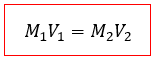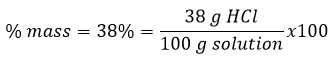# Problem: Concentrated HCl has a density of 1.19 g/mL and is 38.0% by mass HCl (the rest of the mass is water). How many mL of this concentrated solution do you need to add to a 250.0 mL volumetric flask to create a 0.0500 M HCl solution? Give your answer to the hundredths decimal place and do not include any units. The MW of HCl is 36.46 g/mol.

###### FREE Expert Solution

We’re asked to find the volume in mL of a concentrated HCl solution with a 38% by mass in HCl needed to prepare a 0.0500 M HCl solution from a 250 mL volumetric flask.

Since we’re adding water to a concentrated solution of HCl and diluting it, we need the dilution equation:Where V1 is the initial volume we’re asked to find

M1 is the initial concentration of HCl

M2 is the final concentration of 0.0500 M of HCl

V2 is the final volume of 250 mL.

We need to determine M1 in order to solve for V1 with the dilution equation.

We know the concentrated HCl solution has a percent mass, which is:Where we assume the total mass is 100 g of the solution made between HCl and water, of which 38 g are of HCl.

83% (100 ratings)###### Problem Details

Concentrated HCl has a density of 1.19 g/mL and is 38.0% by mass HCl (the rest of the mass is water). How many mL of this concentrated solution do you need to add to a 250.0 mL volumetric flask to create a 0.0500 M HCl solution? Give your answer to the hundredths decimal place and do not include any units. The MW of HCl is 36.46 g/mol.